# Schrödinger equation facts for kids

Kids Encyclopedia FactsSchrödinger's equation inscribed on the gravestone of Annemarie and Erwin Schrödinger. (Newton's dot notation for the time derivative is used.)

The Schrödinger equation is a differential equation (a type of equation that involves an unknown function rather than an unknown number) that forms the basis of quantum mechanics, one of the most accurate theory of how subatomic particles behave. It is a mathematical equation that was thought of by Erwin Schrödinger in 1925. It defines a wave function of a particle or system (group of particles) which has a certain value at every point in space for every given time. These values have no physical meaning (in fact, they are mathematically complex), yet the wave function contains all information that can be known about a particle or system. This information can be found by mathematically manipulating the wave function to return real values relating to physical properties such as position, momentum, energy, etc. The wave function can be thought of as a picture of how this particle or system acts with time and describes it as fully as possible.

The wave function can be in a number of different states at once, and so a particle may have many different positions, energies, velocities or other physical property at the same time (i.e. "be in two places at once"). However, when one of these properties is measured it has only one specific value (which cannot be definitely predicted), and the wave function is therefore in just one specific state. This is called wave function collapse and seems to be caused by the act of observation or measurement. The exact cause and interpretation of wave function collapse is still widely debated in the scientific community.

For one particle that only moves in one direction in space, the Schrödinger equation looks like: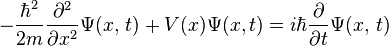$-\frac{\hbar^2}{2m}\frac{\partial^2}{\partial x^2}\Psi(x,\,t) + V(x)\Psi(x,t) = i\hbar\frac{\partial}{\partial t} \Psi(x,\,t)$

where$i$ is the square root of -1,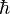$\hbar$ is the reduced Planck's constant,$t$ is time,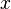$x$ is a position,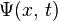$\Psi(x,\,t)$ is the wave function, and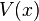$V(x)$ is the potential energy, an as yet not chosen function of position. The left hand side is equivalent to the Hamiltonian energy operator acting on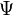$\Psi$.

## Time independent Version

Assuming that the wave function,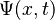$\Psi (x,t)$, is separable, i.e. assuming the function of two variables can be written as the product of two different functions of a single variable: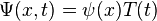$\Psi (x,t) = \psi (x) T(t)$

then, using standard mathematical techniques of Partial differential equations, it can be shown that the wave equation can be rewritten as two distinct differential equations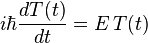$i\hbar \frac{d T(t)}{dt} = E \, T(t)$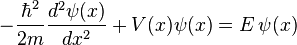$-\frac{\hbar^2}{2m} \frac{d^2 \psi (x)}{dx^2}+V(x) \psi (x) = E \, \psi (x)$

where the first equation is solely dependent on time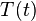$T(t)$, and the second equation depends only on position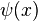$\psi (x)$, and where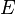$E$ is just a number. The first equation can be solved immediately to give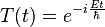$T(t) = e^{-i\frac{Et}{\hbar}}$

where$e$ is Euler's number. Solutions of the second equation depend on the potential energy function,$V(x)$, and so cannot be solved until this function is given. It can be shown using quantum mechanics that the number$E$ is actually the energy of the system, so these separable wave functions describe systems of constant energy. Since energy is constant in many important physical systems (for example: an electron in an atom), the second equation of the set of separated differential equations presented above is often used. This equation is known as the Time independent Schrödinger Equation, as it does not involve$t$.

## Interpretations of the Wave function

### Born Interpretation

There are many philosophical interpretations of the wave function, and a few of the leading ideas will be considered here. The main idea, called the Born probability interpretation (named after physicist Max Born) comes from the simple idea that the wave function is square integrable; i.e.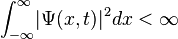$\int_{-\infty}^\infty \! |\Psi (x,t)|^2 dx < \infty$

This rather simple formula has great physical implications. Born hypothesized that The above integral determines that the particle exists somewhere in space. But how can we find it? We use the integral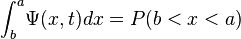$\int_b^a \! \Psi (x,t) dx=P(b

where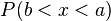$P(b is the probability of finding the particle in the region from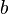$b$ to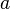$a$. In other words, all that can be known in advance about a particle in general are probabilities, averages, and other statistical quantities associated with its physical quantities (position, momentum, etc.). Basically, this is the Born interpretation.

### Copenhagen Interpretation

An extension of the above ideas can be made. Since the Born interpretation says that the actual position particle cannot be known, we can derive the following. If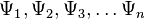$\Psi_1, \Psi_2, \Psi_3, \dots \Psi_n$ are solutions to the wave equation, then the superposition of those solutions, i.e.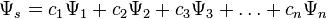$\Psi_s=c_1\Psi_1 + c_2\Psi_2 + c_3\Psi_3 + \dots + c_n\Psi_n$

is also a solution. This implies, then, that the particle exists in every possible position. When an observer comes and measures the position of the particle, then the superposition is reduced to a single possible wave function. (i.e.,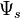$\Psi_s$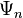$\Psi_n$, where$\Psi_n$ is any of the possible wave function states.) This idea that a particle's position cannot exactly be known, and that a particle exists in multiple positions simultaneously gives rise to the Uncertainty principle. The mathematical formulation of this principle can be given by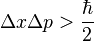$\Delta x\Delta p>\frac{\hbar}{2}$

Where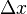$\Delta x$ is the uncertainty in position, and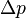$\Delta p$ is the uncertainty in momentum. This principle can be mathematically derived from the Fourier transforms between momentum and position as defined by quantum mechanics, but we will not derive it in this article.

### Other Interpretations

There are various other interpretations, such as the many-worlds interpretation, and quantum determinism.

## Images for kidsSchrödinger equation Facts for Kids. Kiddle Encyclopedia.